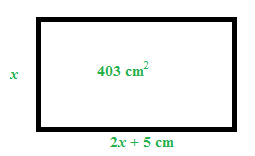Home > CCAA8 > Chapter cca9 > Lesson cca9.1.3 > Problem9-30

9-30.

Solve the following problem using any method. Write your solution as a sentence.

The length of a rectangle is $5$ cm longer than twice the length of the width. If the area of the rectangle is $403$ square centimeters, what is the width? Homework Help ✎

Make a diagram and label the sides.

Write an equation to solve for the width ($x$).

Distribute and solve for $x$.

Use the quadratic formula to solve for $x$.

$x=\frac{52}{4}=13 \;\;\;\;\;\;\;\;\; x=\frac{-62}{4}=-15.5$

Can't have a negative length.$(2x+5)(x)=403$

$2x^2+5x=403$
$2x^2+5x−403=0$

$x=\frac{-5\pm\sqrt{5^{2}-4(2)(-403)}}{2(2)}$

$x=\frac{-5+57}{4} \; \text{and} \; x=\frac{-5-57}{4}$